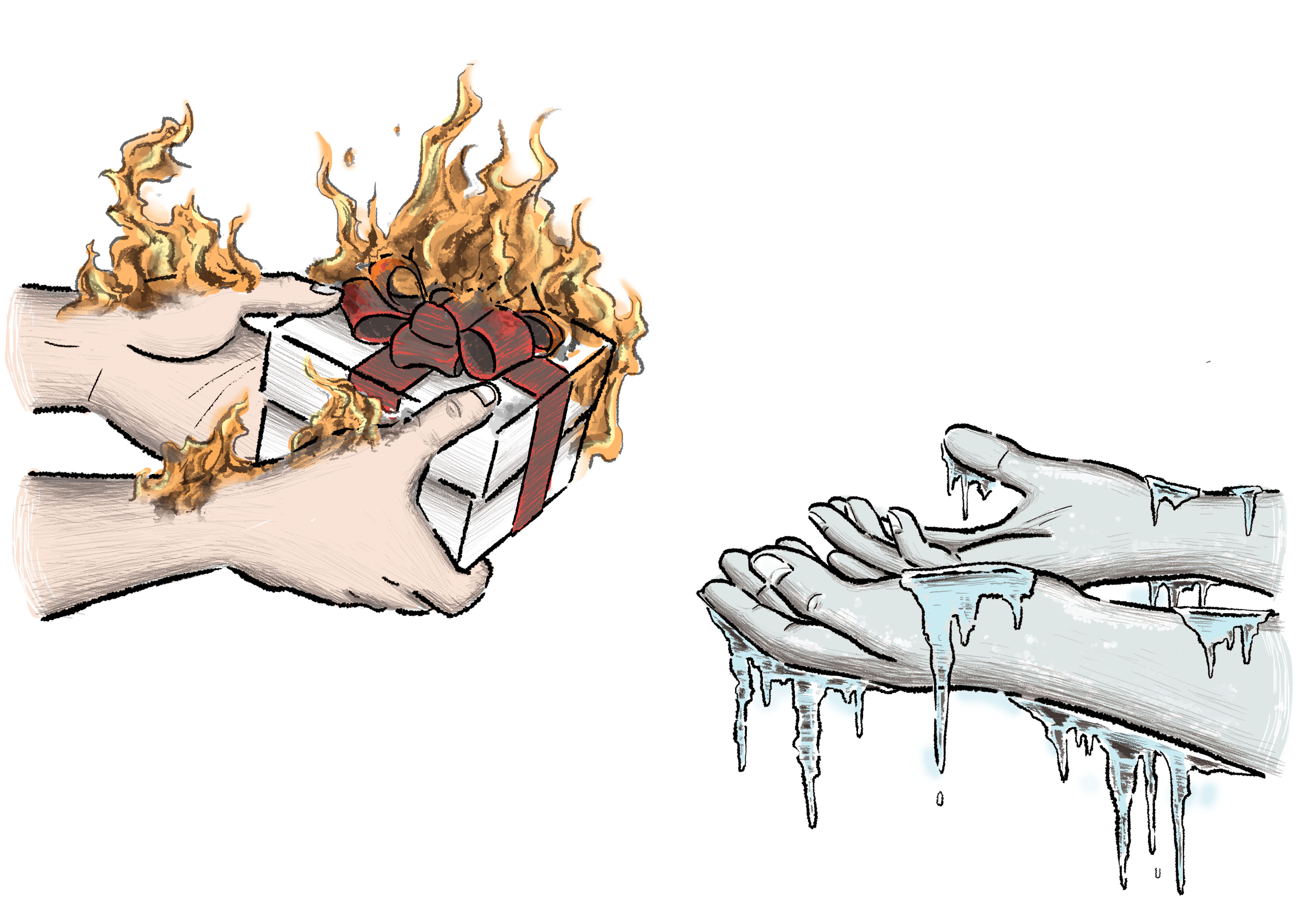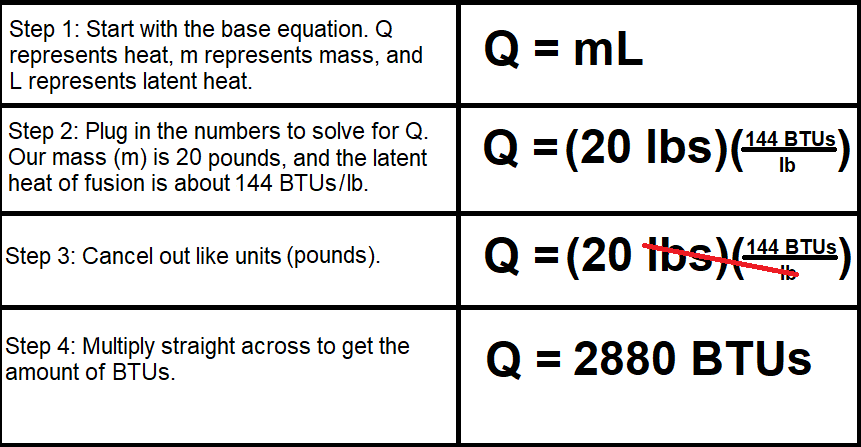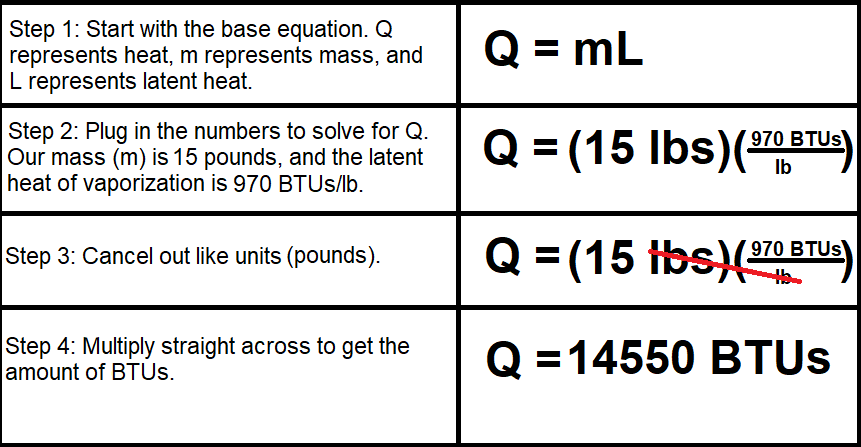## Understanding Heat TransferHeat is a byproduct of mechanical energy that presents itself as a temperature increase. French physicist James Prescott Joule aimed to equate heat to mechanical energy. Early experiments used paddle-like structures to stir water within a container and raise its temperature. The paddles would move and agitate the water whenever a mass on a pulley system would fall from a fixed height. Joule evaluated the relationship between the energy he put in and the temperature increase.

He created an equation that multiplies all the variables of potential energy to determine the heat produced (in Joules, J). These variables are mass in kilograms (m), the force of gravity (g = 9.8 m/s2), and height in meters (h).

mgh = J

If a 10 kg mass were to fall 1 meter, then the equation would look like this:

10 kg(9.8 m/s2)(1 m) = J

The resulting number is 98 Joules, but this is not sufficient to raise the temperature of water significantly. Another equation equates heat (Q) to the mass (m) of water multiplied by temperature change (ΔT) and the amount of energy required to raise the temperature of a given substance (coefficient of heat, c).

Q = cmΔT

After further experimentation, Joule determined that it takes 4186 Joules to raise the temperature of a kilogram of water by 1° Celsius. This is the coefficient of heat (c) for water.##### Units of Heat

There are three main units for measuring heat: calories, Joules, and British Thermal Units (BTU).

A calorie (cal) is the amount of heat required to raise the temperature of 1 gram of water from 14.5° Celsius to 15.5° Celsius (ΔT = 1° Celsius). The temperature change (ΔT) is only equivalent to 1° Celsius with a starting temperature (T0) of 14.5° Celsius and final temperature (TF) of 15.5° Celsius. However, these are the standard temperatures used to define a calorie. These calories are not the same as the calories we consume in our diets; the calories we consume are kilocalories (kcal), equivalent to 1000 calories.

A Joule (J) is the standard unit of energy named after the previously mentioned physicist, James Prescott Joule. A single calorie is equivalent to 4.186 Joules. Since we often use kilograms to measure the mass of water, it is worth noting that it takes 4186 Joules to increase a single kilogram of water by 1° Celsius.

A British Thermal Unit (BTU) is the amount of heat required to raise the temperature of 1 pound of water from 63° Fahrenheit to 64° Fahrenheit (ΔT = 1° Fahrenheit). Notice that BTUs use the imperial system while calories and Joules use the SI (or metric system). Even though BTUs are not very common internationally, many American heaters and air conditioning units are still rated in BTUs. One BTU per pound Fahrenheit is equal to one calorie per gram Celsius, but one BTU per pound Celsius is equivalent to about 1.8 calories per gram Celsius.

##### Heat CapacityHeat capacity is the ability of an object to store heat. An object’s mass and specific heat primarily influence its heat capacity.

It takes more heat to raise the temperature of an object with a greater mass. Let’s say you have two objects of the same material, but one of them is ten times larger than the other. Intuitively, it would take ten times the amount of heat to raise the larger object’s temperature by the same amount as the smaller object. The larger object can store more heat without increasing the temperature, giving it a higher heat capacity than the smaller object.

Specific heat is the amount of heat required to raise the temperature of the unit mass of a substance by 1° Celsius. Substances have different specific heats, which affects the heat capacity of each substance. For example, a pound of water has a greater heat capacity than a pound of copper. Even though you have the same amount of each substance, water’s specific heat is about ten times greater than copper’s. It takes ten times the amount of heat to cause water’s temperature to increase the same amount as copper’s. In the equation, Q = cmΔT, the coefficient of heat (c) represents the specific heat of the measured substance. Figure 1 shows a table of different substances and their specific heats.Figure 1. The specific heats of water, aluminum, copper, glass, and lead. Each column represents the specific heats in different units: BTUs per pound Fahrenheit, BTUs per pound Celsius, calories per gram Celsius, and Joules per kilogram Celsius. Notice that the BTUs per pound Fahrenheit are equivalent to the calories per gram Celsius.

##### Heat and Temperature Increase

You can use the equation Q = cmΔT to measure a change in temperature if you already know the substance’s initial temperature, mass, and how much energy is being added. We would rewrite the equation as Q = cm(TF – T0), where TF represents the final temperature and T0 represents the initial temperature.

Since we know every variable except TF, we are going to solve for TF. To isolate TF, divide both sides of the equation by cm, and add T0 to both sides. The new equation looks like Q/cm + T0 = TF. Divide Q by cm to get the temperature change, and add that quotient to the initial temperature (T0) to get the new temperature (TF). See Figure 2 for a step-by-step visualization of this process.Figure 2. The step-by-step mathematical process for finding the final temperature after applying 50 BTUs of heat to 5 pounds of water at 15° Celsius.

##### Phase Changes and Latent HeatWhether a solid becomes a liquid or a liquid changes to a gas, phase changes require energy. The added energy changes a substance’s molecular structure. The energy stimulates movement, which reconfigures the molecular bonds. When a solid melts, the vibrations force the molecules to fall away from each other and slide past one another. When a liquid turns into a gas, the molecules’ movements become so fast and powerful that they break free of the liquid and become a gas or vapor.

It takes more energy to cause a phase change than to heat a substance. It takes 1 BTU to heat 1 pound of water, but it takes about 144 BTUs to melt 1 pound of ice completely. Evaporating liquid water takes even more energy; boiling water transforms into water vapor after adding 970 BTUs.

The temperature does not change during a phase change. An ice cube at 32° Fahrenheit (0° Celsius) will transform into water at 32° Fahrenheit. Because there is no temperature change, we do not use TF – T0 to account for the energy change. Instead, we use a fixed variable for latent heat. Latent heat is the heat required for a substance to undergo a phase change, not change temperature. There are two kinds of latent heat: latent heat of fusion and latent heat of vaporization. Figure 3 shows the heat energy applied to water and the corresponding temperatures.Figure 3. The heat energy applied to water (in BTUs) and the corresponding temperatures. Notice that the temperature stays the same at the melting and boiling points of water. The energy added during these phase changes is latent heat.

Latent heat of fusion is the amount of energy required for a substance to change from a liquid to a solid or vice versa (via freezing or melting). Waters latent heat of fusion is roughly equivalent to 144 BTUs per pound. Figure 4 shows how to calculate the number of BTUs needed to melt an ice cube that weighs 20 pounds.Figure 4. Solving for Q using the 20-pound ice cube and water’s latent heat of fusion.

Latent heat of vaporization is the amount of energy needed for a substance to change from a liquid to a gas or vice versa (via evaporation or condensation). Waters latent heat of vaporization at atmospheric pressure is equivalent to 970 BTUs per pound. Figure 5 shows how to calculate the number of BTUs needed to vaporize boiling water with a weight of 15 pounds.Figure 5. Solving for Q using the 15-pound mass and water’s latent heat of vaporization.

##### Heat Transfer and Refrigerants

The HVAC industry relies on refrigerants to remove heat. Refrigerants are substances that move heat through an HVAC system’s cycle and produce a cooling effect while vaporizing. Refrigerants exist in liquid and gaseous states when they cycle through a unit, so a refrigerant’s specific heat and latent heat of vaporization affect its performance.

There are a few variables to consider when choosing a refrigerant in a cycle with changing temperatures and pressures. A good refrigerant has pressures that can be easily manipulated. Since gases are much more compressible than liquids, the boiling point of a good refrigerant should be below the temperature of the room or refrigerated box. Although the boiling point should be low when the refrigerant is in the evaporator, its condensation point should be above the outdoor temperature. The refrigerant must be able to reject the outdoor heat.

Since a refrigerant’s goal is to move heat, an ideal refrigerant should have a high latent heat of vaporization. When a refrigerant has a high latent heat of vaporization, it can effectively move more heat when it boils and condenses within the target ranges.

If you intend to use a refrigerant as a pump fluid in the liquid phase, it would be best for the refrigerant to have a relatively high specific heat. Water and carbon dioxide are common refrigerants that we use as pump fluids in their liquid phases. If you look at Figure 6, you can see that water and carbon dioxide have much higher specific heats than other common refrigerants. Keep in mind that we do not commonly use the other refrigerants as pump fluids in the liquid phase.*Water is listed at 212 degrees F at 14.7 PSIA rather than a 40-degree evaporator temperature.

Figure 6. The specific heats and latent heats of vaporization of common refrigerants compared to water.

Water may seem like the ideal refrigerant based on the previous information about specific heat and latent heat of vaporization, but it has several drawbacks. Refrigerants come into contact with metals during the refrigeration cycle, so nonreactive refrigerants are best for HVAC systems. Water has oxygen in it, which reacts with many metals and will most likely harm an HVAC system. Water would also require evaporators to be in a carefully controlled deep vacuum. Since this is highly impractical and inefficient, we rely on other refrigerants that don’t need the evaporator to be under such demanding conditions constantly.

When it comes to other refrigerants, you have to consider toxicity and flammability. Toxicity refers to a refrigerant’s potential to cause injury via a corrosive attack on the bodily tissues. ASHRAE classifies refrigerants into two toxicity categories: Class A and Class B. Class A refrigerants are primarily non-toxic. Most of the refrigerants in Figure 6 are Class A refrigerants. Class B refrigerants are toxic and should be handled with care. ASHRAE also has a classification system for a refrigerant’s flammability, the ease with which a refrigerant combusts. There are three categories for flammability: No Flame Propagation (1), Lower Flammability (2 and subclass 2L, which represents the slow burn in the new HFOs), and Higher Flammability (3). R-32 and R-290 are part of Class 3, presenting a high fire risk and requiring extreme caution.

ASHRAE classifies each refrigerant with the letter for toxicity and the number for flammability. For example, R-22 is an A1 refrigerant because it is non-toxic and has no risk of flame propagation. A1 is the best category for a refrigerant because A1 refrigerants present a low risk for bodily harm or property damage.

One more thing to consider when selecting a refrigerant is how it will mix with oil. Since oil will have to circulate through the system, it will come into contact with the refrigerant at some point. The oil should be able to move and mix with the refrigerant well, meaning that the oil should have good miscibility.

This is all to say – moving heat can be a tricky business, but it’s one we are excited to tackle.

This site uses Akismet to reduce spam. Learn how your comment data is processed.

Scroll to top
Translate »

### Daily Tech Tip

Get the (near) daily Tech Tip email right in your inbox!